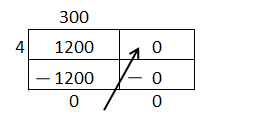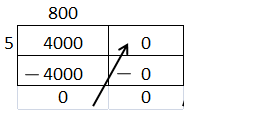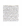# Texas Go Math Grade 4 Lesson 9.3 Answer Key Divide Tens, Hundreds, and Thousands

Refer to our Texas Go Math Grade 4 Answer Key Pdf to score good marks in the exams. Test yourself by practicing the problems from Texas Go Math Grade 4 Lesson 9.3 Answer Key Divide Tens, Hundreds, and Thousands.

## Texas Go Math Grade 4 Lesson 9.3 Answer Key Divide Tens, Hundreds, and Thousands

Essential Question

How can you divide numbers through thousands by whole numbers through 10?
Divide using basic facts and place value,
thousands will convert in to hundreds by dividing whole number through 10.

Unlock the Problem.Dustin is packing apples in gift boxes. Each gift box holds 4 apples. How many boxes can Dustin pack with 120 apples?

You can divide using basic facts and place value.
30 boxes
Explanation:
Each gift box holds 4 apples.
Dustin has 120 apples
Number of boxes Dustin pack
120 ÷ 4 = 30

Example 1 Divide. 120 ÷ 4

STEP 1 Identify the basic fact.
12 ÷ 4
12 ÷ 4 = 3

STEP 2 Use place value. 120 = _____________ tens
12 tens

STEP 3 Divide.
12 tens ÷ 4 = ___________ tens ← Think: 4 × 3 tens = 12 tens
= _____________
120 ÷ 4 = 30
So, Dustin can pack _____________ boxes.

Example 2 Divide. 1,200 ÷ 4
STEP 1 Identify the basic fact.
12 ÷ 4
12 ÷ 4 = 3

STEP 2 Use place value. 1,200 = _____________ hundreds
3 hundreds

STEP 3 Divide.
12 hundreds ÷ 4 = _____________ hundreds ← Think: 4 × 3 hundreds = 12 hundreds
= _____________
1,200 ÷ 4 = 300
Explanation:
1,200 ÷ 4 = 300Explain how to use a basic fact and place value to divide 4,000 ÷ 5.
Explanation:
4000 ÷ 5 = 800Math Talk

Mathematical Processes
Describe the pattern in the place value of the dividends and quotients.
Use place value understanding and properties of operations to perform multi-digit arithmetic. Fluently add and subtract multi-digit whole numbers using the standard algorithm.

Share and Show

Question 1.
Divide. 2,800 ÷7
What basic fact can you use? ______________
2,800 = 28 ______________
28 hundreds ÷ 7 = ______________
2,800 ÷ 7 = ______________
2,800 ÷ 7 = 400
What basic fact can you use
28 ÷ 7
2,800 = 28 hundreds
28 hundreds ÷ 7 = 400
Explanation:
Step I: Identify the basic fact.
Step II: Use place value.
Step III: Divide

Go Math Lesson 9.3 Go Math 4th Grade Question 2.
Divide. 280 ÷ 7
What basic fact can you use?
280 = 28 ______________
28 tens ÷ ______________ = 4 ______________
280 ÷ 7 = ______________
What basic fact can you use
280 ÷ 10
280 = 28 tens
28 tens ÷ 7 = 4 tens
280 ÷ 7 = 40
Explanation:
Step I: Identify the basic fact.
Step II: Use place value.
Step III: Divide

Math Talk

Mathematical Processes
Explain how Exercises 1 and 2 alike and different.
Both exercises 1 and 2 are alike.
Explanation:
In exercise 1 place value is hundreds and,
in exercise 2 place value is thousands.

Use basic facts and place value to find the quotient.

Question 3.
360 ÷ 6 = ___________
Explanation:
basic fact can you use
36 ÷ 6
360 = 36 tens
36 tens ÷ 6 = 6 tens
360 ÷ 7 = 60

Question 4.
2,000 ÷ 5 = ___________
Explanation:
basic fact can you use
20 ÷ 5
2,000 = 20 hundreds
20 hundreds ÷ 5 = 4 hundreds
2,000 ÷ 7 = 400

Question 5.
4,500 ÷ 9 = ___________
Explanation:
basic fact can you use
45 ÷ 9
4,500 = 45 hundreds
45 hundreds ÷ 9 = 5 hundreds
4,500 ÷ 9 = 500

Problem Solving

Algebra Find the unknown number.

Question 6.
420 ÷= 60
Explanation:
Let X be unknown number
420 ÷ X = 60
42 tens ÷ 7 = 6 tens
420 ÷ 7 = 60

Question 7.÷ 4 = 30 .
120 ÷ 4 = 30
Explanation:
Let be unknown number
÷ 4 = 30
= 30 x 4
= 120

Question 8.
810 ÷= 90
Explanation:
Let X be unknown number
810 ÷ X = 90
81 ÷ X = 9
X = 9
810 ÷ 9 = 90

Question 9.
H.O.T. Divide 400 ÷ 40. Explain how patterns and place value can help.
Explanation:
basic fact can you use
40 ÷ 4
400 = 40 tens = 4 hundreds
40 tens ÷ 4 = 10
400 ÷ 10 = 40

Question 10.
Multi-Step In 3 minutes, a damseifly’s wings beat 2,700 times and a butterfly’s wings beat 2,100 times. About how many more times did the damseifly’s wings beat in one minute than did the butterfly’s wings?
Explanation:
In 3 minutes, a damseifly’s wings beat 2,700 times
In 1 minute, a damseifly’s wings beat 2,700 ÷ 3 = 900
In 3 minutes, a butterfly’s wings beat 2,100 times.
In 1 minute, a butterfly’s wings beat 2,100 ÷ 3 = 700
About how many more times did the damseifly’s wings beat in one minute than the butterfly’s wings
900 – 700 = 200 times.

Problem Solving

Question 11.
Jamal put 600 pennies into 6 equal rolls. How many pennies were in each roll?
100 pennies
Explanation:
Jamal put 600 pennies into 6 equal rolls.
Number of pennies were in each roll
600 ÷ 6 = 100

Sela has 6 times as many coins now as she had 4 months ago. If Sela has 240 coins now, how many did she have 4 months ago?
Explanation:
Sela has 6 times as many coins now as she had 4 months ago.
If Sela has 240 coins now,
Number of coins she had 4 months ago
240 x 6 = 1440

Question 13.H.O.T. Multi-Step Chip collected 2,090 dimes. Sue collected 1,910 dimes. They divided all their dimes into 8 equal stacks. How many dimes are in each stack?
500 dimes.
Explanation:
Chip collected 2,090 dimes.
Sue collected 1,910 dimes.
Total dimes together 2,090 + 1,910 = 4000
They divided all their dimes into 8 equal stacks.
Number of dimes in each stack
4000 ÷ 8 = 500 dimes.

Question 14.
Communicate Mr. Roberts sees a rare 1937 penny. The cost of the penny is $210. If he saves$3 a week, will Mr. Roberts have enough money to buy the penny in one year? Explain.
No, Mr.Robert doesn’t have enough money to buy.
Explanation:
Mr. Roberts sees a rare 1937 penny.
The cost of the penny is $210. If he saves$3 a week
In one year he saves 52 x 3 = $156 ( 1 year has 52 weeks ) 210 – 156 =$54 less

Question 15.
H.O.T. Multi-Step Analyze Mrs. Roberts sold each of 5 coins for the same dollar amount.She received a total of $300. Each coin cost her$32. How much money did she make on each coin? Explain how you got your answer.
$28 on each coin. Explanation: Mrs. Roberts sold each of 5 coins for the same dollar amount. She received a total of$300.
Each coin cost her $32. Total cost of 5 coins = 32 x 5 =$160
Total money she make on each coin
300 – 160 = 140
140 ÷ 5 = 28

Question 16.
Jeremy has a shelf that is 150 centimetres long. If each DVD case is 3 centimetres wide, how many DVD cases can fit on the shelf?
(A) 50
(B) 5
(C) 45
(D) 30
Option(A)
Explanation:
Jeremy has a shelf that is 150 centimetres long.
If each DVD case is 3 centimetres wide,
Number of DVD cases can fit on the shelf
150 ÷ 3 = 50
15 ÷ 3 = 5
50 DVD cases can fit on the shelf

Question 17.
Aaron 480 sports cards. He needs to place the same number each of 6 storage boxes. How many sports cards will storage box?
80 sports cards will storage box
Explanation:
Aaron 480 sports cards.
He needs to place the same number each of 6 storage boxes.
Number of sports cards will storage box
480 ÷ 6
48 tens ÷ 6
80 sports cards will storage box

Question 18.
Multi-Step Suri picks 125 tomatoes and Lynn picks 85 tomatoes. They pack the tomatoes into boxes so there are 7 in each box. How many boxes do they use?
(A) 3
(B) 70
(C) 18
(D) 30
option (D)
Explanation:
Suri picks 125 tomatoes and
Lynn picks 85 tomatoes.
They pack the tomatoes into boxes so there are 7 in each box.
Total tomatoes picked 125 + 85 = 210
7 tomatoes in one box
Number of Boxes required = 210 ÷ 7 = 30

TEXAS Test Prep

Question 19.
Which number sentence is NOT true?
(A) 150 ÷ 5 = 30
(B) 400 ÷ 8 = 500
(C) 4,500 ÷ 9 = 500
(D) 5,600 ÷ 7 = 800
Option(B)
Explanation:
400 ÷ 8 = 500 is wrong
As, 400 ÷ 8 = 50

### Texas Go Math Grade 4 Lesson 9.3 Homework and Practice Answer Key

Question 1.
Divide. 3,600 ÷ 4
What basic fact can you use? ___________
3,600 = 36 ___________
36 hundreds ÷ 4 = ___________
3,600 ÷ 4 = ___________
What basic fact can you use?
3,600 ÷ 4
3,600 = 36 hundreds
36 hundreds ÷ 4 = 9 hundreds
3,600 ÷ 4 = 900
Explanation:
Step I: Identify the basic fact.
Step II: Use place value.
Step III: Divide

Question 2.
Divide. 3,600 ÷ 4
What basic fact can you use? ___________
3,600 = 36 ___________
36 hundreds ÷ ___________ = 9 ___________
3,600 ÷ 4 = ___________
What basic fact can you use?
3,600 ÷ 4
3,600 = 36 hundreds
36 hundreds ÷ 4 = 9 hundreds
3,600 ÷ 4 = 900
Explanation:
Step I: Identify the basic fact.
Step II: Use place value.
Step III: Divide

Use basic facts and place value to find the quotient.

Question 3.
240 ÷ 6 = ___________
Explanation:
basic fact can you use
24 ÷ 6
240 = 24 tens
24 tens ÷ 6 = 4 tens
240 ÷ 6 = 40
Question 4.
3,000 ÷ 6 = ___________
Explanation:
basic fact can you use
30 ÷ 6
3,000 = 30 hundreds
30 hundreds ÷ 6 = 5 hundreds
3,000 ÷ 6 = 500

2,100 ÷ 7 = ___________
Explanation:
basic fact can you use
21 ÷ 7
2,100 = 21 hundreds
21 hundreds ÷ 7 = 3 hundreds
2,100 ÷ 7 = 300

Question 6.
490 ÷ 7 = ___________
Explanation:
basic fact can you use
49 ÷ 7
490 = 49 tens
49 tens ÷ 7 = 7 tens
490 ÷ 7 = 70

Question 7.
5,600 ÷ 8 = ___________
Explanation:
basic fact can you use
56 ÷ 8
5,600 = 56 hundreds
56 hundreds ÷ 8 = 7 hundreds
5,600 ÷ 8 = 700

Question 8.
320 ÷ 4 = ___________
Explanation:
basic fact can you use
32 ÷ 4
320 = 32 tens
32 tens ÷ 4 = 8 tens
320 ÷ 4 = 80

Find the unknown number.

Question 9.
480 ÷= 80 ___________
Explanation:
Let X be unknown number
480 ÷ X = 80
48 ÷ X = 8
X = 48 ÷ 8 = 6
480 ÷ 6 = 80

Question 10.÷ 9 = 50 ___________
Explanation:
Let be unknown number
÷ 9 = 50
= 50 x 9
= 450

Question 11.
640 ÷= 80 ___________
Explanation:
Let X be unknown number
640 ÷ X = 80
64 ÷ X = 8
X = 64 ÷ 8 = 8
640 ÷ 8 = 80

Problem Solving

Question 12.
The sandwich shop delivered 240 box lunches for the high school band. An equal number of boxes will be placed on each of 6 tables. How many boxes will be on each table?
40 b0xes.
Explanation:
The sandwich shop delivered 240 box lunches for the high school band.
An equal number of boxes will be placed on each of 6 tables.
Number of boxes on each table
240÷6 = 40 boxes

Question 13.
Roy bought a package of 500 index cards. He wants to divide the cards into 5 equal stacks. I-low many cards will he in each stack?
500 cards in each stack.
Explanation:
Roy bought a package of 500 index cards.
He wants to divide the cards into 5 equal stacks.
Number of cards in each stack
500÷ 5 = 100

Lesson Check

Question 14.
Which number sentence is not true?
(A) 540 ÷ 9 = 60
(B) 4,900 ÷ 7 = 70
(C) 3,000 ÷ 6 = 500
(D) 1,600 ÷ 8 = 200
Option(B)
Explanation:
4,900 ÷ 7 = 70 is not correct
As, 4,900 ÷ 7 = 700

Question 15.
What number correctly completes the equation?÷ 9 = 800
(A) 72
(B) 720
(C) 7,200
(D) 72,000
Option(C)
Explanation:
Let be unknown number
÷ 9 = 800
= 800 x 9
= 7200

Question 16.
The school office received a shipment of 250 pens. If the shipment was divided equally into 5 boxes, how many pens were in each box?
(A) 250
(B) 25
(C) 5
(D) 50
Option(D)
Explanation:
The school office received a shipment of 250 pens.
If the shipment was divided equally into 5 boxes,
Total pens in each box
250 ÷ 5 = 50

Go Math Grade 4 Practice and Homework Lesson 9.3 Answer Key Question 17.
City workers counted how many large trucks passed over a bridge in one week. If 3,500 trucks pass over the bridge in one week, how many trucks pass over the bridge each day?
(A) 700
(B) 70
(C) 500
(D) 50
Option(C)
Explanation:
City workers counted how many large trucks passed over a bridge in one week.
If 3,500 trucks passed over the bridge in one week,
Total trucks passed over the bridge each day
3500÷7 = 500 ( 1 week has 7 days )

Question 18.
Multi-Step Eileen collected 98 empty cans to recycle and Carl collected 82 cans. They packed an equal number of cans into three boxes to take to the recycling center. How many cans were in each box?
(A) 60
(B) 6
(C) 40
(D) 4
Option(A)
Explanation:
Eileen collected 98 empty cans to recycle and
Carl collected 82 cans.
Total cans together 98+82 = 180
They packed an equal number of cans into three boxes to take to the recycling center.
180÷3=60
Number of cans in each box 60

Question 19.
Multi-Step A baker sold 8 cakes for the same dollar amount. He received a total of $240. Each cake cost the baker$18 to make. How much money did he make on each cake?
(A) $30 (B)$12
(C) $21 (D)$13
He received a total of $240. Each cake cost the baker$18 to make.
Total cost of 8 cakes = 18 x 8 = $144 Total money he makes on each cake 240 – 144 =$96